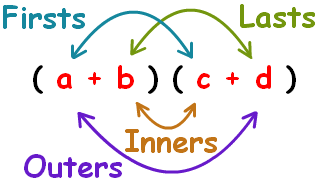Give us feedbackX

# FOIL Calculator

23 Sep 2015Homework Help Online

## What is Foil Calculator?

Foil stands for:

F = First
O = Outer
I = Inner
L = Last

It is the method used to perform multiplication. It finds the product of the two terms. It gives the result instantly by using the Foil Calculator.

## This explains the foil method:Steps to use the Foil method by Foil Calculator:

1. Firstly, multiply the first terms of both the brackets.
2. Then multiply the first term with the last term of the first and the second bracket respectively.
3. Next step is to multiply the second term of the first bracket with the first term of the second bracket.
4. At last, multiply the last terms of both the brackets.
5. Now, add all the answers and we attain the final result.

Apply Foil rule to multiply the terms in a specific order that is First, Outside, Inside, Last. Foil method is used to multiply two binomial equations and not for polynomials. For example:

Multiply the two binomials using FOIL method,

(3x-4) and (2x+1)

1. Multiply the first terms: (3x) x (2x) = 6 x2
2. Multiply the outside terms: (3x) x (1) = 3x
3. Multiply the inside terms: (-4) x (2x) = -8x
4. Multiply the last terms: (-4) x (1) = -4

Binomial equation = (6x2 + 3x - 8x – 4) = 6x2 _ 5x - 4

Examples:

1. (2x + 3)(3x + 4)

Step 1:  2x × 3x = 6X2
Step 2: 2x × 4 = 8x
Step 3:  3 × 3x = 9x
Step 4:  3 × 4 = 12
Step 5:  6x2 + 8x + 9x + 12.
Answer:  6x2 + 17x + 12

1. A university student designed a rectangular picture frame. The picture measures 17 cm by 11 cm. The total area of the picture frame is 315 cm2. Find the width of the frame.

Step 1:  Let x be the width of the frame.
Length and Width of the picture frame are 17 + 2x and 11 + 2x respectively.
Step 2:  Since area of picture frame = 315 cm2
=> (17 + 2x)(11 + 2x) = 315  (Because area of rectangle = Length * Width)
Step 3:  17(11 + 2x) + 2x(11 + 2x) = 315 (Foil method)
187 + 34x + 22x + 4x2 = 315
4x2 + 56x + 187 = 315
4x2 + 56x - 128 = 0
4(x + 16)(x - 2) = 0  (Factorized)
x + 16 = 0 and x - 2 = 0
x = -16 and x = 2
Step 4:  Length can't be negative. So our value is x = 2.
Step 5:  Width of the frame is 2 cm.

Uses of Foil Calculator:

• It is the method to calculate the binomial product of any expression
• It gives instant results
• It’s an easy tool
• In addition, it is not time consuming
• The method is not difficult to learn
• Just remember the Foil rule: first, outside, inside, last

The Foil method is an easy method to perform the multiplications just by following the steps irrespective of the numbers, whether it’s a fraction or a negative sign.

http://calculator.tutorvista.com/foil-calculator.html

This website shows the working of the Foil calculator. Also the image available on the site elaborates the method of the calculator.

http://www.polymathlove.com/polymonials/midpoint-of-a-line/online-calculator-with-foil.html

http://www.algebra.com/algebra/homework/Distributive-associative-commutative-properties/foil.solver

Here you can learn about the method of Foil calculator by putting the figures of your choice. It helps you to learn how to expand the two binomials.

http://www.freemathhelp.com/using-foil.html

Free math help is a very basic site to understand the method or you can say it is for the beginners. To start with, you may visit this website.

This site is also useful for reading and analyzing more problems related to Foil calculator.

http://www.mathwarehouse.com/algebra/polynomial/foil-method-binomials.php

Free math help is a very basic site to understand the method or you can say it is for the beginners. To start with, you may visit this website.>

https://www.easycalculation.com/algebra/multiply-binomials-foilmethod.php

Here online calculator is accessible to solve your problems on Foil calculator.

Related Posts

Testimonials

• Jason L.

I have a lot of questions that need to be answered. While searching online, I found the site of StudyFAQ and it is all worth it. The tutor is very kind and I’ve got everything I needed. Thank you so much.

• Garry T.

Tutor did a great job. The process was very fast and efficient. I didn’t know that there is a quality and reliable tutoring service provider online. I can now start with my assignment.

• Margret O.

I was really having a hard time finding an expert who can help me with my topic. Thanks to StudyFAQ.com, I can start working on my assignment now.